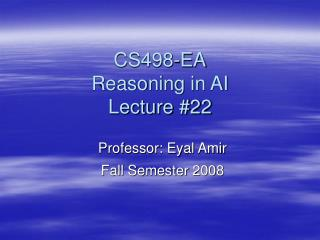DownloadDownload PresentationCS498-EA Reasoning in AI Lecture #22

# CS498-EA Reasoning in AI Lecture #22

Télécharger la présentation## CS498-EA Reasoning in AI Lecture #22

- - - - - - - - - - - - - - - - - - - - - - - - - - - E N D - - - - - - - - - - - - - - - - - - - - - - - - - - -
##### Presentation Transcript

1. CS498-EAReasoning in AILecture #22 Professor: Eyal Amir Fall Semester 2008

2. Probabilistic Modal Logic Afsaneh Shirazi (speaker) Eyal Amir University of Illinois at Urbana-Champaign

3. Motivation • Poker (all kinds of games that need combination of probability + knowledge) Alice Bob What does Bob have?

4. Problem Definition • How to represent probabilistic belief about probabilistic belief? • How to reason in this representation? • Solution: adding probability to modal logic (probabilistic modal logic), providing reasoning algorithms

5. Outline • Probabilistic modal logic • Probabilistic knowledge • Reasoning algorithms • Top-Down • Bottom-Up • Sampling subgraphs (approximate) • Experimental results Exact

6. Modal Logic & Probability • Kripke strusture in modal logic: • Set of states • Accessibility relation • Interpretation True state of the world We add probability to accessibility relation

7. Probabilistic Modal Logic • Set of states • Probabilistic accessibility relation • Interpretation P(S2|S1) S2 S1 P(S1|S1) P(Sn|S1) Sn

8. Syntax • Example: (K1(w1) < .5)  w2 • Logical connectives are: • X • X  Y • Ki(X) < N where N is [0 1] • Ki(X) = N • For each modal function we have different probabilistic accessibility relation

9. Outline • Probabilistic modal logic • Probabilistic knowledge • Reasoning algorithms • Top-Down • Bottom-Up • Sampling subgraphs (approximate) • Experimental results Exact

10. Probabilistic Knowledge • The probabilistic accessibility relation is an equivalence relation An equivalence class for player 1

11. Outline • Probabilistic modal logic • Probabilistic knowledge • Reasoning algorithms • Top-Down • Bottom-Up • Sampling subgraphs (approximate) • Experimental results Exact

12. Reasoning Algorithms • Evaluate queries about a given state • Example: K1(K2(w2) < 1/4) > 2/3 • Alice (player 1) knows her hand and the board cards, she knows her equivalence class • Question: Is the query true on a given state?

13. Outline • Probabilistic modal logic • Probabilistic knowledge • Reasoning algorithms • Top-Down • Bottom-Up • Sampling subgraphs (approximate) • Experimental results Exact

14. K2 S’1 S’m Top-Down (ToDo) • K1(K2(w2) < 1/4) > 2/3 on state S • Compute the value of the query recursively S1 S K1 Sn

15. Top-Down (ToDo) • K1(K2(w2) < 1/4) > 2/3 on state S • Compute the value of the query recursively S1 S K1 We compute the value of K2(w2)<¼ for all states accessible from S Sn

16. Top-Down • K1(K2(w2) < 1/4) > 2/3 on state S • Compute the value of the query recursively • Complexity: O(|q| nm) |q|: query size m: # of nested modal function n: # of states accessible from a state

17. Outline • Probabilistic modal logic • Probabilistic knowledge • Reasoning algorithms • Top-Down • Bottom-Up • Sampling subgraphs (approximate) • Experimental results Exact

18. Bottom-Up (BU) • K1(K2(w2) < 1/4) > 2/3 on state S • We compute K2(w2 ) < 1/4 on all states (rename it as t) • We compute K1(t) > 2/3 on S • Complexity: O (|q| |S|) |S|: total number of states • When the number of nested modal functions grows, Bottom-Up is faster

19. Outline • Probabilistic modal logic • Probabilistic knowledge • Reasoning algorithms • Top-Down • Bottom-Up • Sampling subgraphs (approximate) • Experimental results Exact

20. Sampling Subgraphs • Faster than BU when the number of states is large • Faster than ToDo when the branching factor is large • Use sampling to estimate K(x) < r

21. Sampling Subgraphs • Value of K1(w1) < 1/2on s • Number of samples = 3 S1 S Sn

22. Sampling Subgraphs • Value of K1(w1) < 1/2on s • Number of samples = 3 S1 S w1=0 w1=1 Si w1=1 Sn

23. Transforming to a Bayesian Network • q = K1(w1) < 1/2on s • We compute P(q = 1|w1(s)=1, w1(s1)=0, w1(si)=1) • This value is an estimate of the value of the query 1 0 1 q K1(w1) < 1/2

24. Outline • Probabilistic modal logic • Probabilistic knowledge • Reasoning algorithms • Top-Down • Bottom-Up • Sampling subgraphs (approximate) • Experimental results Exact

25. Experimental Results

26. Conclusion & Future Work • We provide: • Syntax and semantics for probabilistic modal logic • Exact and approximate reasoning methods to evaluate a query • Approximate method is only faster when we have very large structure • Future work: belief update, inference and learning in this model

27. THE END